RADAR speed detectors bounce microwave radiation off of moving vehicles and detect the reflected waves. These waves are shifted in frequency by the Doppler effect, and the beat frequency between the directed and reflected waves provides a measure of the vehicle speed.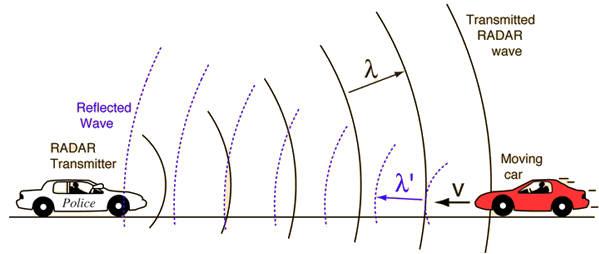Speed detection calculation
Index

Traveling wave concepts

 HyperPhysics***** Sound R Nave
Go Back

# Doppler Shift, Moving Target

The Doppler shift for relatively low velocity sources such as those encountered by police RADAR is given by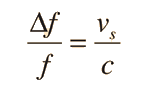where c is the speed of light (and all electromagnetic waves in a vacuum). But in this case there are two shifts: one because the wave incident on the moving car is Doppler shifted and an additional shift because the reflection is from a moving object. The frequency shift of the reflected wave received at the source of the wave is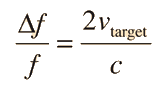This shift is detected by measuring the beat frequency with the transmitted wave.Derivation Calculation of wavelength Calculation of frequency Doppler effect for light
Index

Traveling wave concepts

Doppler concepts

 HyperPhysics***** Sound R Nave
Go Back

# Beat Frequency and Speed

The beat frequency between a microwave transmitted signal and a reflected signal off a moving object is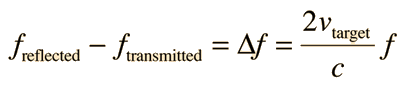where the target velocity is taken as positive if the target if approaching the transmitter. Police RADAR uses this method for measurement of auto speed.Calculation of speed
Index

Traveling wave concepts

Doppler concepts

 HyperPhysics***** Sound R Nave
Go Back

# Beat Frequency and Speed

For a transmitted frequency of GHz = Hz

the reflected frequency will be Hz

and the beat frequency will be Hz

if the speed of the vehicle is vtarget = m/s = mi/hr = km/hr = ft/s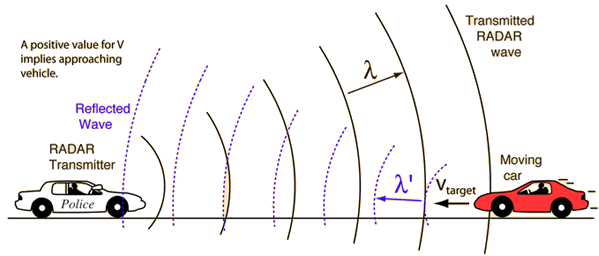Note: If not entered as data, the transmitted frequency will default to 10.6 GHz, a typical X-band radar frequency. K-band units with frequencies on the order of 30 GHz are also used. Any parameters can be changed.

 Police RADAR Expressions used in calculation Doppler effect for electromagnetic waves
Index

Traveling wave concepts

Doppler concepts

 HyperPhysics***** Sound R Nave
Go Back